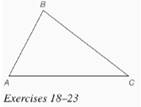Chapter 7.CR, Problem 20CRElementary Geometry For College St...

7th Edition
Alexander + 2 others
ISBN: 9781337614085

Solutions

Chapter
SectionElementary Geometry For College St...

7th Edition
Alexander + 2 others
ISBN: 9781337614085
Textbook Problem

In Review Exercises 18 to 23, use construction methods with the accompanying figure. Given: Δ A B CFind: The orthocenter

To determine

To find: The orthocenter of the triangle ABC.

Explanation

Given:

The triangle ABC is shown below.

Definition used:

Orthocenter:

The intersection of any two altitudes determines the orthocenter of a triangle.

Calculation:

Consider the triangle ABC.

First construct the altitude from A to BC¯.

Draw an arc from A to intersect BC¯ at D and E.

Now draw equal arcs from D and E to intersect at X

Still sussing out bartleby?

Check out a sample textbook solution.

See a sample solution

The Solution to Your Study Problems

Bartleby provides explanations to thousands of textbook problems written by our experts, many with advanced degrees!

Get Started

Change 40 mi/h to ft/s.

Elementary Technical Mathematics

10. What is the mean of the scores in Problem 8?

Mathematical Applications for the Management, Life, and Social Sciences

Evaluate the integral. 67. 1x+1+xdx

Single Variable Calculus: Early Transcendentals

sinx=ex+ex2

Study Guide for Stewart's Single Variable Calculus: Early Transcendentals, 8th